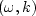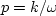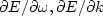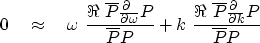Next: TOMOGRAPHY AND OTHER APPLICATIONS Up: A FULLY TWO-DIMENSIONAL PE Previous: The hope method

## An alternative principle for 2-D interpolation

In principle, missing traces can be determined to simplify-space. Consider a wave field P composed of several linear events in (t,x)-space. A contour plot of energy in-space would show energy concentrations along lines of various,much like Figure 18. Let the energy density be.Along contours of constant E, we should also see.The gradient vector ()is perpendicular to the contours. Thus the dot product of the vectorwith the gradient should vanish. I propose to solve the regression that the dot product of the vectorwith the gradient of the log energy be zero, or, formally,(14)
The variables in the regression are the values of the missing traces. Obviously, the numerator and the denominator should be smoothed in small windows in the-plane. This makes conceptual sense but does not fit well with the idea of small windows in (t,x)-space. It should be good for some interesting discussions, though. For example, in Figure 18, what will happen where event lines cross? Is this formulation adequate there? Also, how should the Nyquist limitation on total bandwidth be expressed?Next: TOMOGRAPHY AND OTHER APPLICATIONS Up: A FULLY TWO-DIMENSIONAL PE Previous: The hope method
Stanford Exploration Project
10/21/1998